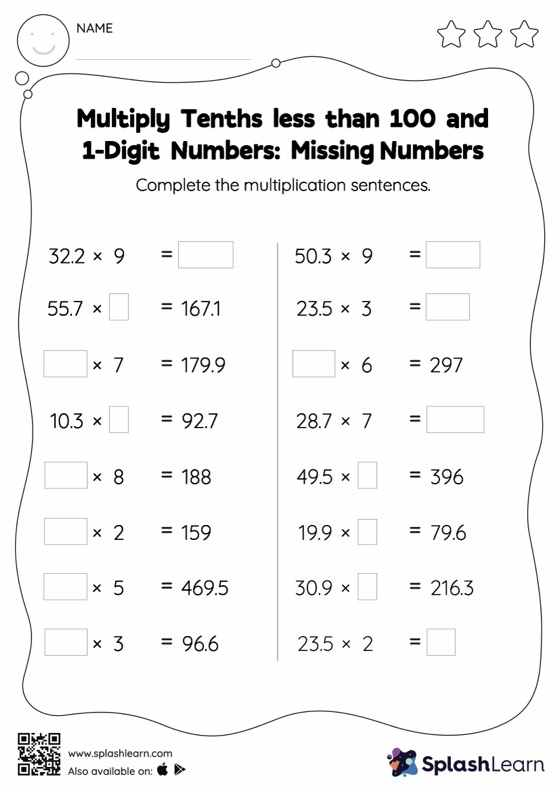# Multiply Tenths less than 100 and 1-Digit Numbers: Missing Numbers Worksheet

Home > Multiply Tenths less than 100 and 1-Digit Numbers: Missing NumbersDoes your child know how to multiply tenths less than 100 and 1-digit numbers? Invite them to practice this concept here. As the worksheet uses a horizontal format, it allows for more creativity when it comes to how the student solves the problem. In contrast, in the vertical format, students usually employ the standard method to solve.